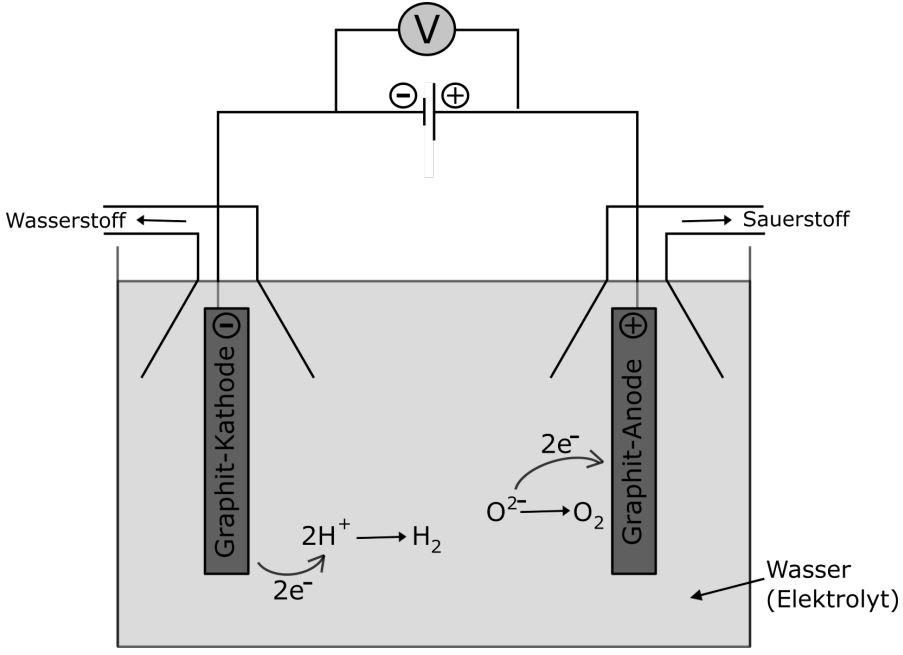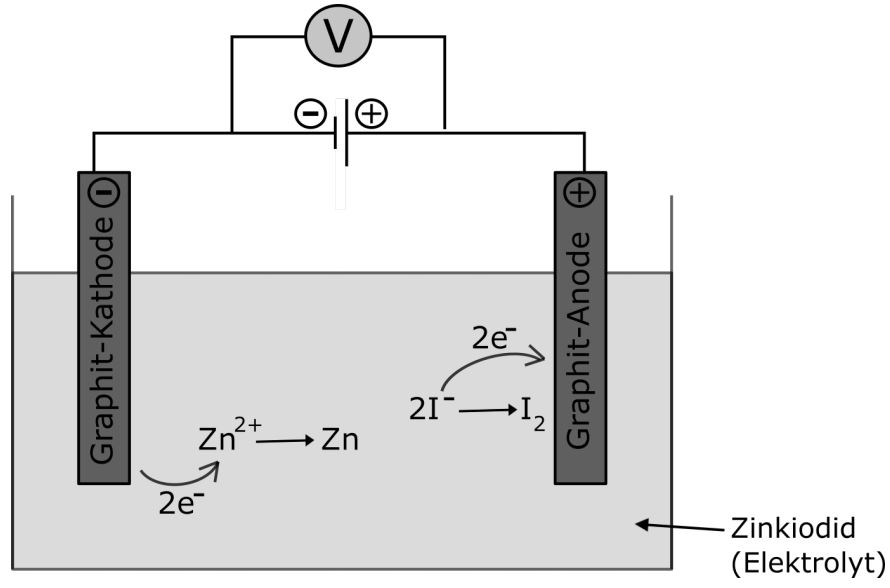# What is the name of an electrolytic cell

## electrolysis

Electrolysis is a process in which an electric current creates a redox reaction. In this article, we'll take a closer look at the reactions that take place in electrolysis:The water particles are broken down into oxygen and hydrogen with the help of electricity. Let's look at the reactions that take place at the cathode and anode. At the cathode, water reacts to form hydrogen (this is a reduction, since the oxidation number of the hydrogen atom in the water molecule is + I and in the hydrogen molecule is 0). At the anode, water reacts to form oxygen (this is an oxidation because the oxidation number of the oxygen atom in the water molecule is -II and in the oxygen molecule is 0).

\ begin {array} {llcl}
Cathode / Red .: & {2H_2O} \ + \ {2e ^ {-}} \ quad \ rightarrow \ quad {H_2} \ + \ {2OH ^ {-}} \
Anode / Ox .: & {2H_2O} \ quad \ rightarrow \ quad \ {O_2} + \ {4H ^ {+}} \ + \ {4e ^ {-}}
\ end {array}

So that we can now combine the two reactions into one overall reaction, the number of electrons in both partial equations must again agree. The reaction at the cathode must therefore be multiplied by two:

\ begin {array} {llcl}
\ text {Cathode / Red .:} & {2H_2O} \ + \ {2e ^ {-}}; \ quad \ rightarrow \ quad {H_2} + \ {2OH ^ {-}} \ quad | \ cdot 2 \
& {4H_2O} \ + \ {4e ^ {-}} \ quad \ rightarrow \ quad \ {2H_2} \ + \ {4OH ^ {-}}
\ end {array}

Now we can combine the two partial equations into one overall equation:

\ begin {align *}
{4H_2O} \ + \ {4e ^ {-}} \ + \ {2H_2O} \ quad \ rightarrow \ quad {2H_2} \ + \ {4OH ^ {-}} \ + \ {O_2} \ + \ {4H ^ {+}} \ + \ {4e ^ {-}}
\ end {align *}

Now the electrons are struck out:

\ begin {align *}
{4H_2O} \ + \ {2H_2O} \ quad \ rightarrow \ quad {2H_2} \ + \ {4OH ^ {-}} \ + \ {O_2} \ + \ {4H ^ {+}}
\ end {align *}

Electrolysis is a chemical reaction in which electrical energy is used to force a redox reaction. A chemical compound is broken down in the process. Electrical energy is converted into chemical energy.

Electrolysis is also the process that takes place when a battery is charged. In principle, this is the inversion of a galvanic element. The structure of an electrolysis is very similar to that of a galvanic element: two electrodes are immersed in a solution, the electrolyte. Then, unlike the galvanic element, direct current is applied, which means that we have a plus and a minus pole. For example, consider a zinc iodide solution with two graphite electrodes:The negative electrode attracts positive particles, in this case the Zn2 + ions. Electrons are transferred from the electrode to the zinc (II) ions, which creates elemental zinc. The positive electrode attracts negative particles, in this case the I ions. These can now deliver their electrons to the anode, creating elemental iodine.

Now here is not only zinc iodide, but also water, which can be decomposed to hydrogen and oxygen by electrolysis. How can we now decide which of the two electrolyses to run? Here we can again use the electrochemical series of voltages. The electrolysis of substances with smaller potentials always takes place first.

Let's compare zinc iodide and water:

\ begin {array} {l | l | l}
\ text {Electrolysis of} \ dots & \ text {reaction equations} & \ text {standard potential} \
\ hline
\ dots \ text {Water} & \ {2H_2O} \ + \ {2e ^ {-}} \ quad \ rightarrow \ quad {H_2} \ + \ {2OH ^ {-}} \ & \ text {-0.83 } \ {V} \
& \ {O_2} \ + \ {4H ^ {+}} \ + \ {4e ^ {-}} \ quad \ rightarrow \ quad {2H_2O} & \ text {+1.23} \ {V} \
\ hline
\ dots zinc iodide & \ {Zn ^ {2+}} \ + \ {2e ^ {-}} \ quad \ rightarrow \ quad {Zn} & \ text {-0.76} \ {V} \
& \ {2I ^ {-}} \ quad \ rightarrow \ quad {I_2} \ + \ {2e ^ {-}} \ & +0 {,} 53 \ {V} \
\ end {array}

The standard potentials for the decomposition of zinc iodide are smaller in terms of amount than those for the decomposition of water. Therefore, the electrolysis of zinc iodide occurs first.

If we want to make sure that only the electrolysis of zinc iodide is taking place, we can also apply a low voltage so that it is just sufficient to decompose zinc iodide. How high does this tension have to be?

We calculate this again as with the galvanic cell - the smaller standard potential is subtracted from the larger standard potential:

\ begin {align *}
0.53 V - (−0.76 V) = +1.29 V
\ end {align *}

This means that we apply a voltage of 1.29 V, which is just enough to decompose zinc iodide, but not for the electrolysis of water (a voltage of at least 2.06 V would have to be applied here).

Now we come to a special phenomenon in electrolysis. We already know how to calculate the voltage necessary for the electrolysis to take place. Sometimes nothing happens when the calculated voltage is applied. Electrolysis only takes place at a higher voltage. The voltage that has to be applied in addition to the calculated value is called overvoltage or overpotential.

This overvoltage depends on various factors:

• Type and surface properties of the electrode
• Type and concentration of ions
• Temperature and current density

If metals are produced during electrolysis, the overvoltage is lower than in electrolysis, which produces gases.